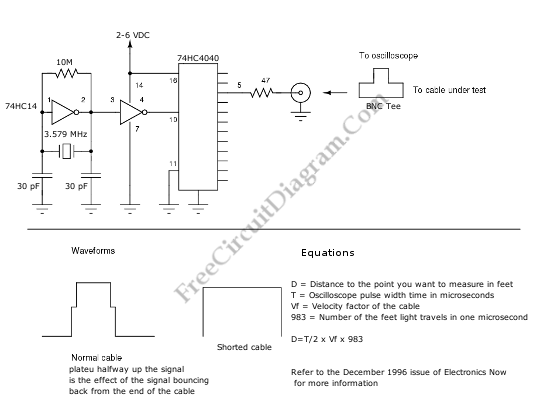# Cable Reflection TesterThis is a Cable Reflection Tester circuit. This circuit can be used to check coax cable runs for shorts or even impedance mismatches. This circuit will send a pulse down the circuit, then the return signal can be checked on an oscilloscope. The distance can be determined by using this equation:
distance = time x speed equations.

Here is the schematic diagram of the circuit :By determining the distance, the circuit can check coax cable runs for shorts or impedance mismatches. Make sure the time is divided by 2, and take in account the cable’s velocity factor.